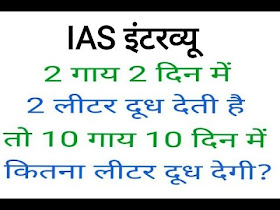## Friday 3 September 2021

### IAS Exam Question ::: 2 cows 2 days gives 2 litre milk ,how much milk gives 10 cows in 10 days

IAS Exam Question ::: 2 cows 2 days gives 2 litre milk ,how much milk gives 10 cows in 10 days2 cows-----2days-------2 litre
1 cow-------2 days-------2/2 litre
10 cows-------1day--------(1×10/2) litre
10 cows-------10 days----5×10 litre=50 litre

2 cows 2 litre 2 day
10 cows x litre 10 days

2 * 2 * x = 10 * 2 * 10
4x = 200

x = 50

Give the date and month of birth of any person

Kids, how fun it is to know this ????

Let's see

Tell someone it's her

(1) Multiply the month of birth by two (2) Add five to the number obtained now (3) Multiply the number obtained now by five

And finally put zero behind the number found. Add your date of birth.

Tell you the number you get and you can tell their date and month of birth.

how???? Let's see

Suppose my date of birth is 19th September.

So I will say September is the 8th month..I will multiply it by two.

Now I will add five to it, that is 3.12 + 2 = 2

Now I will multiply it by five, that is 3.2x2 = 114

And finally I put zero, that's એટલે 1150

I will add my date of birth 19 to it. So 3.1150 + 12 = 119… is equal to ???? Let me tell you the number 115 so what do you do ???

Subtracting 20 from that means 115-40 = 1115. The last two digits (19) are my date of birth.

Now subtract two from the remaining number (11). So 11-9 = 2 is the month of my birth. (Ninth month is September)

My date of birth is 15th September.

Let's look at another example.

One person says the last number is 8.

So what do we do ???

Subtract 50 from 2… 2-20 = 2

The last two digits are their date of birth… ૨૪ and subtract two from the remaining number… 2-5 = ૨ ..this is the month of their birth (April

So you can tell right away that your date of birth is April 8th.

Try it and surprise everyone by using this game when relatives, friends gather on any occasion